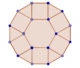Discussion about math, puzzles, games and fun.   Useful symbols: ÷ × ½ √ ∞ ≠ ≤ ≥ ≈ ⇒ ± ∈ Δ θ ∴ ∑ ∫  π  -¹ ² ³ °

You are not logged in.

## #1 2019-07-11 13:26:43

!nval!d_us3rnam3
MemberRegistered: 2017-03-18
Posts: 46

### My Final Vector/Polygon Question

A 5-sided polygon $ABCDE$ is inscribed in a circle centered at the origin. Define the lines
\begin{align*}
\ell_{ABC} &= \text{Line through the centroid of $\triangle ABC$ perpendicular to $\overline{DE}$},\\
\ell_{BCD} &= \text{Line through the centroid of $\triangle BCD$ perpendicular to $\overline{AE}$}, \\
\ell_{CDE} &= \text{Line through the centroid of $\triangle CDE$ perpendicular to $\overline{AB}$}, \\
\ell_{DEA} &= \text{Line through the centroid of $\triangle DEA$ perpendicular to $\overline{BC}$}, \\
\ell_{EAB} &= \text{Line through the centroid of $\triangle EAB$  perpendicular to $\overline{CD}$}. \\
\end{align*}As we see, these are lines going through the centroid of a triangle formed by three consecutive vertices, perpendicular to the line segment formed by the other two vertices.
Prove that $\ell_{ABC}, \ell_{BCD}, \ell_{CDE},\ell_{DEA}$ and $\ell_{EAB}$ are concurrent, and find the expression for the position vector of the point they all go through.

This problem involves vectors in ways I don't understand. I have no idea how to go about this, can you walk me through how to solve it? Thanks!

Last edited by !nval!d_us3rnam3 (2019-07-12 03:47:08)

"If we wanna be great, we can't just sit on our hands" - 2017 NFL Bears draft

Offline

## #2 2019-07-12 03:59:45

bob bundyRegistered: 2010-06-20
Posts: 8,541

### Re: My Final Vector/Polygon Question

Hi,

EDIT

The more I think about it, the more I think what follows is wrong.  I'll leave it in place as I have proved something, just not your problem.  Sorry.

If E is at 12 o'clock, A at 11.30, B at 11.00, C at 10.00 and D at 08.00, then a perpendicular from C D to X would have to be on DC extended.

My revised approach will be to assume with no loss of generality that the circle has radius 1, in which case the points can have I,j coordinates such as (cosA, sinA) for some angle A.  If this leads anywhere I'll start a new post.

Old version follows

I managed this whilst on a train.  Then I realised there's one element of the proof missing.  Haven't got my geometry program to sort it out but you may well spot what to do  so here goes with the major part.

I'll get the line joining the centroid of ABE, say X, and a point on the line CD, say Y.

I'll skip overlines to save time and use p and q as parameters.

You should already know the centroid , X, is at 1/3(a + b + e).  If not, get the midpoint of BE, M, and hence the equation for AM.  Centroid is one third along the line.

Direction of CD is c - d so the equation of CD is r = c + p(c - d).  Say Y is a point on CD.

Equation of XY is

r = c + p(c-d) + q( 1/3(a + b + e) - c - p( c - d) )

= 1/3(a+ b+ e) + (1+p)(1-q)c -p(1-q)d.

If the concurrent point is on all five lines it must have coefficients that are equal so start by setting the c and d components equal

(1+p)(1-q) = -p(1-q). Implies p = - 1/2 so Y is the midpoint of CD and then set the a,b,e components also equal to this

1/3q = 1/2(1-q) implies q = 3/5

Substituting into the equation for XY gives

r = 1/5(a+b+e+c+d)

If you were to construct the other lines in the same way you'd get the same point each time so this is the concurrent point.

What's missing?  I haven't shown that XY is perpendicular to CD.  Should be easy as Y is the midpoint ... Just need to show XY goes through the centre of the circle.  But I haven't found a value of q to make this happen yet.  Can you?

Bob

Children are not defined by school ...........The Fonz
You cannot teach a man anything;  you can only help him find it within himself..........Galileo Galilei
Sometimes I deliberately make mistakes, just to test you!  …………….Bob BundyOffline

## #3 2019-07-13 13:13:41

!nval!d_us3rnam3
MemberRegistered: 2017-03-18
Posts: 46

### Re: My Final Vector/Polygon Question

Can you help me write it in a more legible way? I'm supposed to explain how to solve this problem, and I can't quite follow your reasoning. The problem looks complete; I don't think anything is missing.

EDIT: never mind, this problem is due and I'll just roll with what you've written. I didn't want to waste your time further.

Last edited by !nval!d_us3rnam3 (2019-07-14 11:28:40)

"If we wanna be great, we can't just sit on our hands" - 2017 NFL Bears draft

Offline